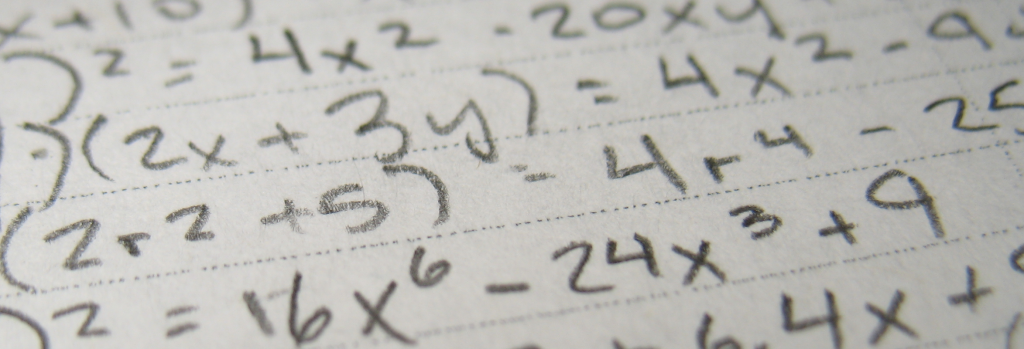Charlottesville
• (434) 296-5111
Great Falls
• (703) 759-3624
McLean
• (703) 288-2808Quadratic equations and factoring are introduced during Algebra I, and they form an important foundation for much of the math students take throughout high school, especially in classes like Algebra II and Precalculus. In my sessions with students from schools like Langley and McLean, factoring is one of the most frequent topics. Students and teachers alike tend to have factoring methods they prefer, which can also add to the confusion. To help clarify this important topic, I wanted to present a few examples of factoring quadratic equations.

I encourage my students to approach regular quadratic equations systematically because this helps them work more efficiently and avoid careless mistakes.

To recap, here are the steps students use when factoring quadratic equations:

Recognize the Equation. Check the format of the equation. Quadratics are generally written in the standard form of Ax2 + Bx + C. When a quadratic meets the following criteria, it can be factored using the steps I demonstrated above:

• Contains three terms
• The first term has a coefficient of 1 and an exponent of 2 (e.g. x2).
• The second term contains a variable and a coefficient (e.g. 3x).
• The third term is an integer (e.g. 42, -10, etc.).

Identify the Answer Format. Regular quadratic equations will fit a consistent format: (x + D) (x + E), where D and E are factors of the constant term C and add to the value of B. I recommend that students set up the answer format before they start calculating because it helps them identify what they already know and what they’re trying to find.

List Possible Factors. Next, students should list all of the possible factors of the constant term C. I remind my students to be careful about positives and negatives. They often forget that two negative numbers multiply to equal a positive number, for instance.

Pick the Pair that Works. After listing the factors of C, students need to pick the pair that adds up to B. These will be the factors of the quadratic equation.

## Factoring Difference of Squares Equations

Students who are good at factoring quadratic equations can sometimes get stuck when they encounter less common forms of quadratics. One of these most common types is the difference of squares equation, which fits the format Ax2 – C. I’ve had good results using these methods to factor such equations:

Here’s a summary of how to factor difference of squares quadratics:

Look for Only Two Terms. Once a student knows what to look for, these quadratics get much simpler. Difference of squares quadratics always fit the format Ax2 – C, where A and C are perfect squares (e.g. 1, 4, 9, etc.). Students should be careful, though. In a difference of squares quadratic, the second term must be subtracted from the first term in order to factor correctly.

Identify the Answer Format. Difference of squares quadratics always factor to the format (Hx + J) (Hx – J), where H is the square root of A and J is the square root of C. Once students know how to identify these, it’s as simple as that.

## Using Slide and Divide to Factor

Even students who are experts at factoring quadratic equations are often baffled when they come across more complicated forms where the lead coefficient A is an integer other than 1. There are several ways to approach these problems, but I want to share a popular trick many students call “Slide and Divide”:

Still not quite sure about slide and divide? Here’s a recap of our steps:

Recognize the Slide and Divide Format. When a quadratic in the standard form Ax2 + Bx + C has a coefficient larger than 1, it becomes a candidate for slide and divide.

Multiply A and C. The first step involves multiplying the A value by the C value and then changing A = 1. For instance, 2x2 + 17x + 30 would be transformed to x2 + 17x + 60.

Factor the Regular Expression. Now that our equation resembles a regular quadratic, we can factor using those methods. So x2 + 17x + 60 will factor to (x + 12) (x + 5).

Divide Both Factors by A. Since we originally multiplied C by A, we need to divide A back out of our factors to get our final answer. When we divide our factors (x + 12) (x + 5) by our original A value of 2, we get (x + 12/2) (x + 5/2).

Simplify to Get the Final Answer. After dividing, we need to simplify the fractions to get our final factored form. (x + 12/2) (x + 5/2) becomes (x + 6) (2x + 5).

## Final Notes on Factoring Quadratics

Students should be aware that the methods I demonstrated above are by no means the only ways to factor. But regardless of the method used, I’ve found that students have the best results when they apply a consistent, methodical approach to these important problems. This enables them to master these fundamental skills and build more advanced skills on this solid foundation.

If you need help with factoring, we encourage you to contact us or book a tutoring session. Our tutors love helping students tackle important math concepts like this, and we customize our lessons so the material makes sense alongside what’s presented in class.Matt Mason
Senior Associate Director
Georgetown Learning Centers

There are many great tricks students can use to factor quadratics. Do you have any favorites? Feel free to share them in the comments below!